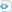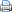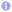C++

# C++11/14 for scientific computing II

## Complex numbers

The template class std::complex and functions for complex numbers (defined in the header file complex) have been extended in C++11/14. The new function std::proj returns the projection of the complex number $z$ onto the Riemann sphere. The functions std::asin, std::acos and std::atan calculate the inverse of the sine, cosine and tangent for complex arguments and similarly std::ahsin, std::ahcos and std::ahtan compute the inverses of the complex hyperbolic trigonometric functions. The member functions real and imag are overloaded in C++11. In C++11 it is not only possible to get the real and the imaginary part of a complex number, the real and the imaginary may now also be set by these functions as illustrated below.

 Source code#include <iostream>
#include <complex>

int main() {
std::complex<double> z;
// set real and imaginary part
z.real(1);
z.imag(2);
// get real and imaginary part
std::cout << "z = " << z.real() << " + " << z.imag() << "i\n";
}

C++14 introduces the literals if, i and id, which represent pure imaginary numbers in single, double and extended precision, respectively. These literals are declared in the inline namespace std::literals::complex_literals and make complex expressions in source code more easy to write and read as the following example shows.

 Source code#include <iostream>
#include <complex>

int main() {
using namespace std::literals;

double pi=std::acos(-1.);
std::complex<double> z=std::exp(1i*pi); // Euler's formula
std::cout << "exp(i, pi) = " << z << '\n';
}